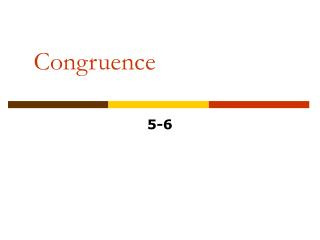DownloadDownload PresentationCongruence

# Congruence

Download Presentation## Congruence

- - - - - - - - - - - - - - - - - - - - - - - - - - - E N D - - - - - - - - - - - - - - - - - - - - - - - - - - -
##### Presentation Transcript

1. Congruence 5-6 Pre-Algebra

2. Warm Up Find the measure of the indicated angle. 1. the fourth angle in a quadrilateral containing angles of 100°, 130°, and 75° 55° 2. the third angle of a right triangle with an angle of 60° 30° 3. the supplement of a 35° angle 145°

3. Vocabulary correspondence

4. A correspondence is a way of matching up two sets of objects. If two polygons are congruent, all of their corresponding sides and angles are congruent. In a congruence statement, the vertices in the second polygon are written in order of correspondence with the first polygon.

5. Example: Writing Congruent Statements Write a congruence statement for the pair of polygons. The first triangle can be named triangle ABC. To complete the congruence statement, the vertices in the second triangle have to be written in order of the correspondence. A@Q, so A corresponds to Q. B@R, so B corresponds to R. C@P, so C corresponds to P. The congruence statement is triangle ABC@ triangle QRP.

6. Example: Writing Congruent Statements Write a congruence statement for the pair of polygons. The vertices in the first pentagon are written in order around the pentagon starting at any vertex. D@M, so D corresponds to M. E@N, so E corresponds to N. F@O, so F corresponds to O. G@P, so G corresponds to P. H@Q, so H corresponds to Q. The congruence statement is pentagon DEFGH@ pentagon MNOPQ.

7. Try This Write a congruence statement for the pair of polygons. The first trapezoid can be named trapezoid ABCD. To complete the congruence statement, the vertices in the second trapezoid have to be written in order of the correspondence. A B | 60° 60° || |||| 120° 120° ||| D C A@S, so A corresponds to S. Q R ||| 120° 120° B@T, so B corresponds to T. || |||| C@Q, so C corresponds to Q. 60° 60° | D@R, so D corresponds to R. T S The congruence statement is trapezoid ABCD@ trapezoid STQR.

8. Try This Write a congruence statement for the pair of polygons. The vertices in the first pentagon are written in order around the pentagon starting at any vertex. 110° A B A@M, so A corresponds to M. 110° 140° 140° F B@N, so B corresponds to N. C 110° C@O, so C corresponds to O. E 110° D N D@P, so D corresponds to P. 110° O M E@Q, so E corresponds to Q. 140° 110° 110° F@L, so F corresponds to L. P 140° L The congruence statement is hexagon ABCDEF@ hexagon MNOPQL. 110° Q

9. WX @ KL a + 8 = 24 –8 –8 a = 16 Example: Using Congruence Relationships to Find Unknown Values In the figure, quadrilateral VWXY@ quadrilateral JKLM. A. Find a. Subtract 8 from both sides.

10. ML @ YX 6b = 30 6b = 30 6 6 Example: Using Congruence Relationships to Find Unknown Values In the figure, quadrilateral VWXY@ quadrilateral JKLM. B. Find b. Divide both sides by 6. b = 5

11. J @V 5c = 85 5c = 85 5 5 Example: Using Congruence Relationships to Find Unknown Values In the figure, quadrilateral VWXY@ quadrilateral JKLM. C. Find c. Divide both sides by 5. c = 17

12. IH @ RS 3a = 6 3a = 6 3 3 Try This In the figure, quadrilateral JIHK@ quadrilateral QRST. A. Find a. Divide both sides by 3. 3a I H a = 2 6 4b° S R 120° J 30° Q K c + 10° T

13. H @S 4b = 120 4b = 120 4 4 Try This In the figure, quadrilateral JIHK@ quadrilateral QRST. B. Find b. Divide both sides by 4. 3a I H b = 30° 6 4b° S R 120° J 30° Q K c + 10° T

14. K @T c + 10 = 30 c + 10 = 30 –10 –10 Try This In the figure, quadrilateral JIHK@ quadrilateral QRST. C. Find c. Subtract 10 from both sides. 3a I H c = 20° 6 90° 4b° S R 120° 90° J 30° c + 10° Q K T

15. Lesson Quiz In the figure, WXYZ@ABCD 10 80° 2. Find mB. 1. Find XY. 8 3. Find CD. 90° 4. Find mZ.